Selina Solutions Concise Mathematics Class 6 Chapter 20 Substitution Exercise 20(A) are well structured by highly experienced faculty having vast knowledge in the education industry. Solutions contain steps to be followed in evaluating the problems relying on algebraic expressions, which is the main concept discussed under this exercise. Practicing solved examples present before the exercise problems help students, in analyzing the question that would appear in the final exam. Students who aspire to obtain proficiency in Mathematics can access Selina Solutions Concise Mathematics Class 6 Chapter 20 Substitution Exercise 20(A) PDF, from the links which are present below.

## Selina Solutions Concise Mathematics Class 6 Chapter 20 Substitution Exercise 20(A) Download PDF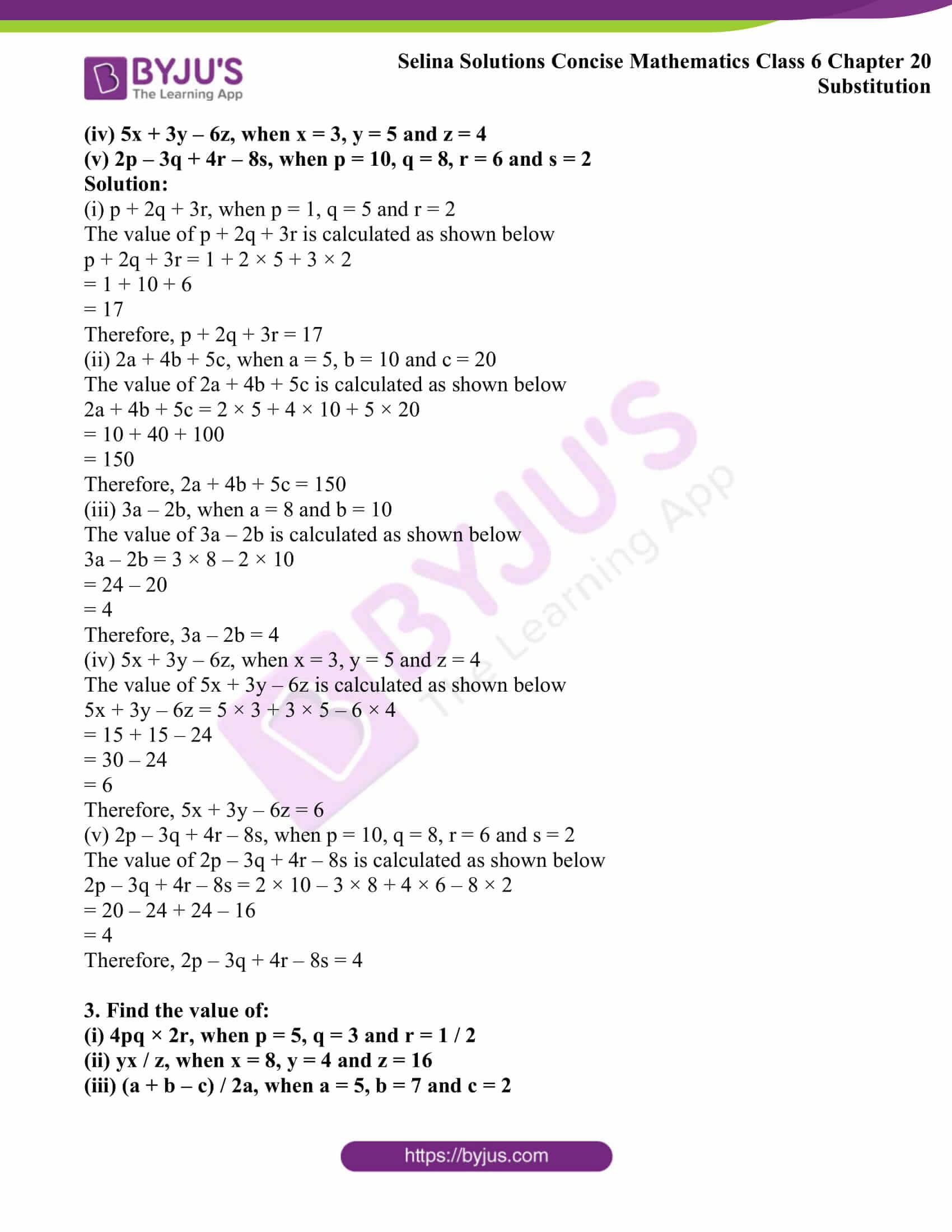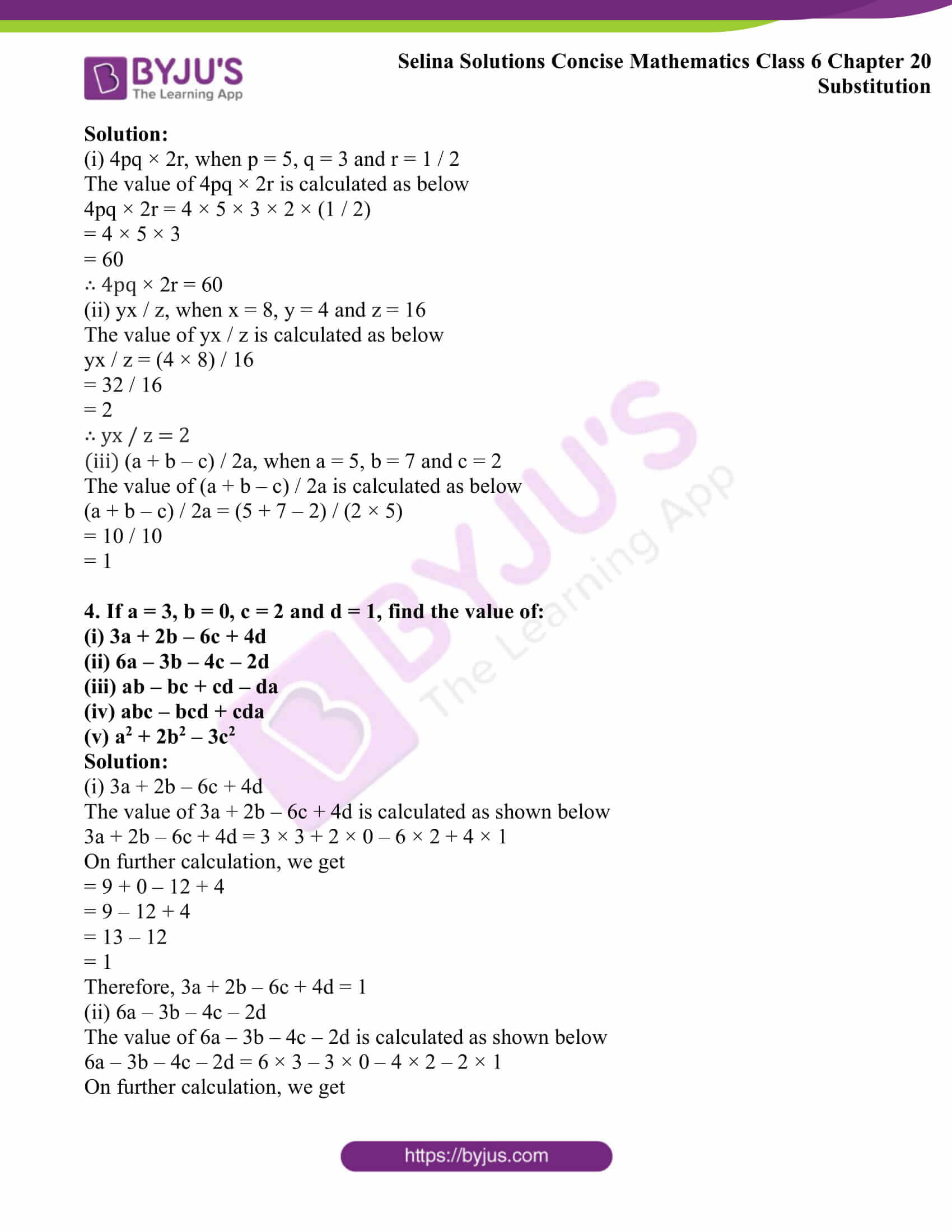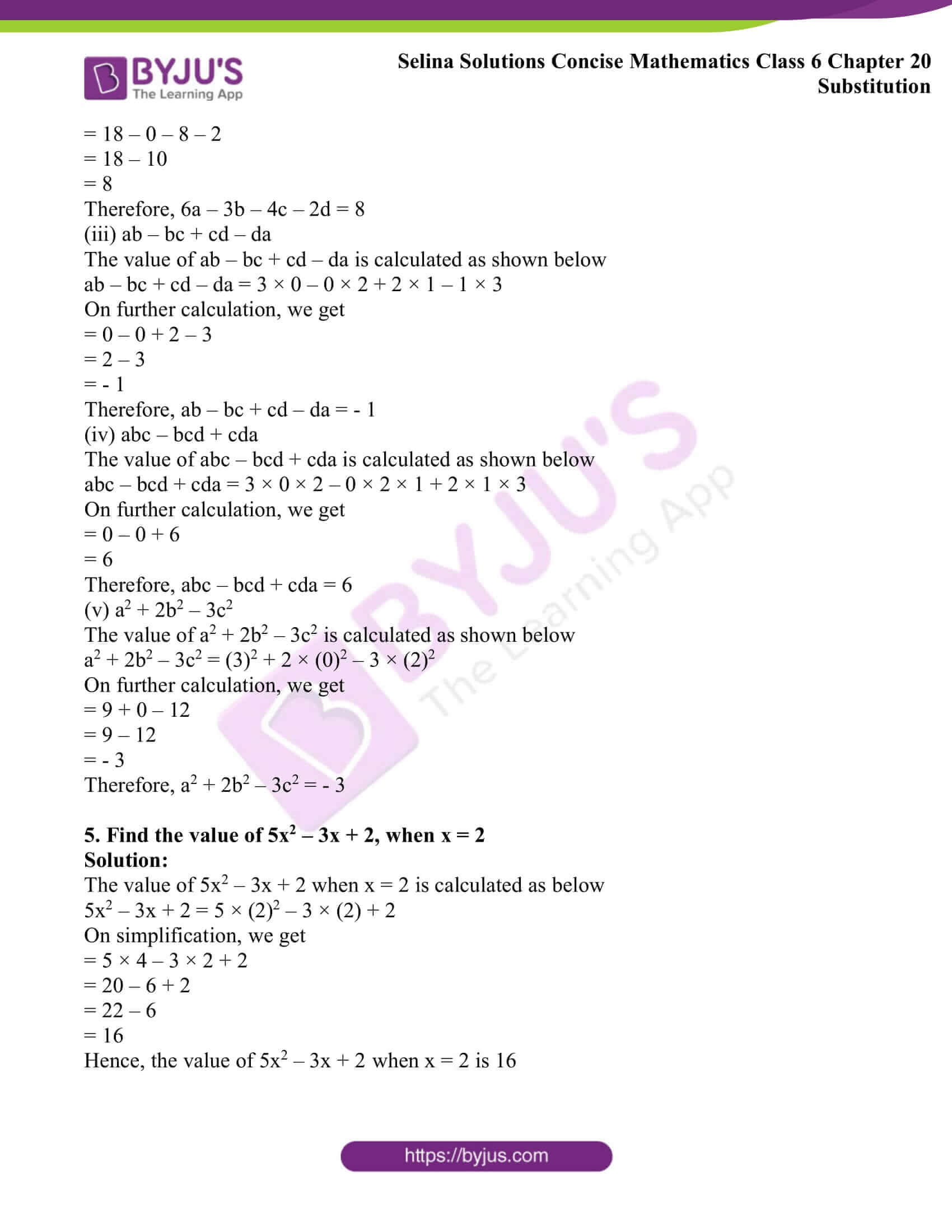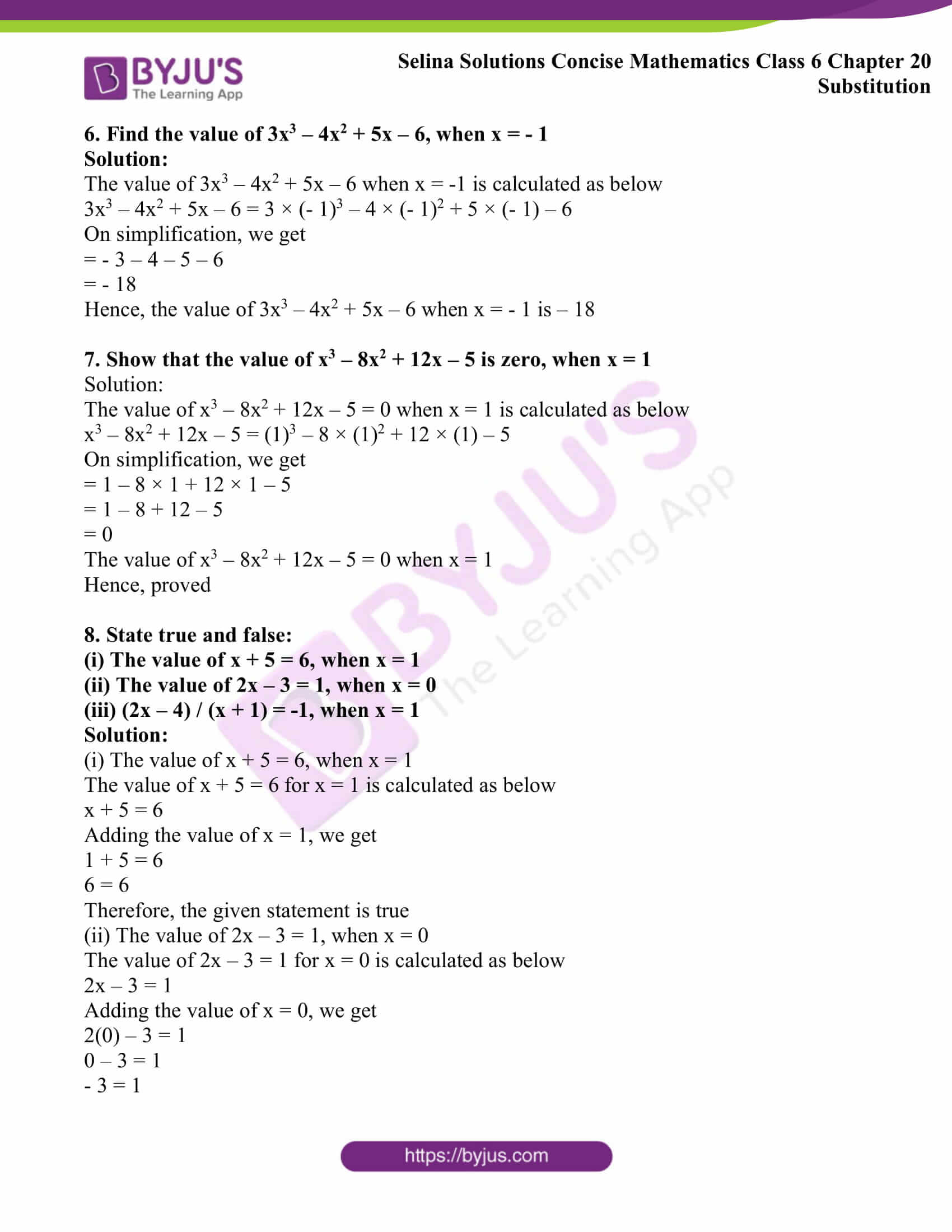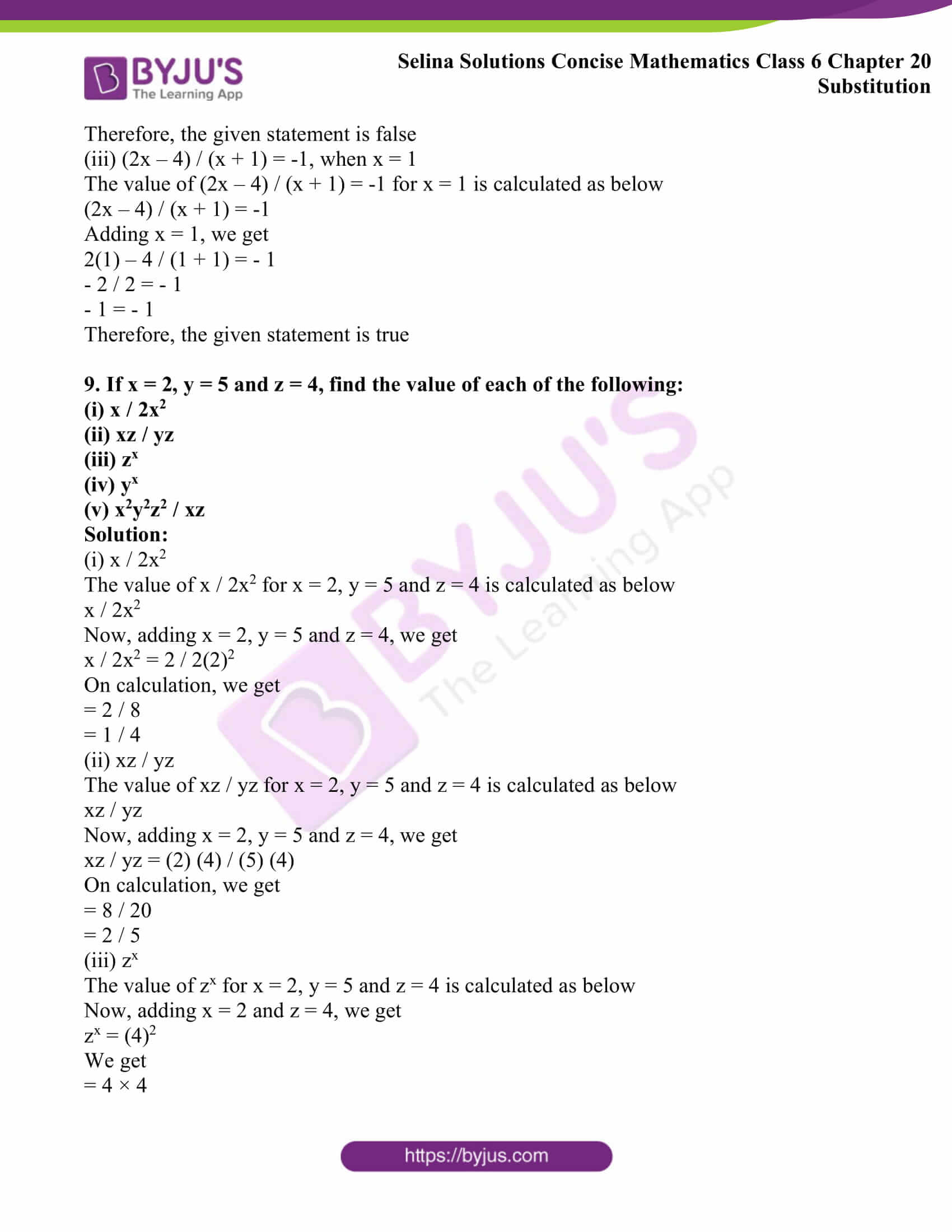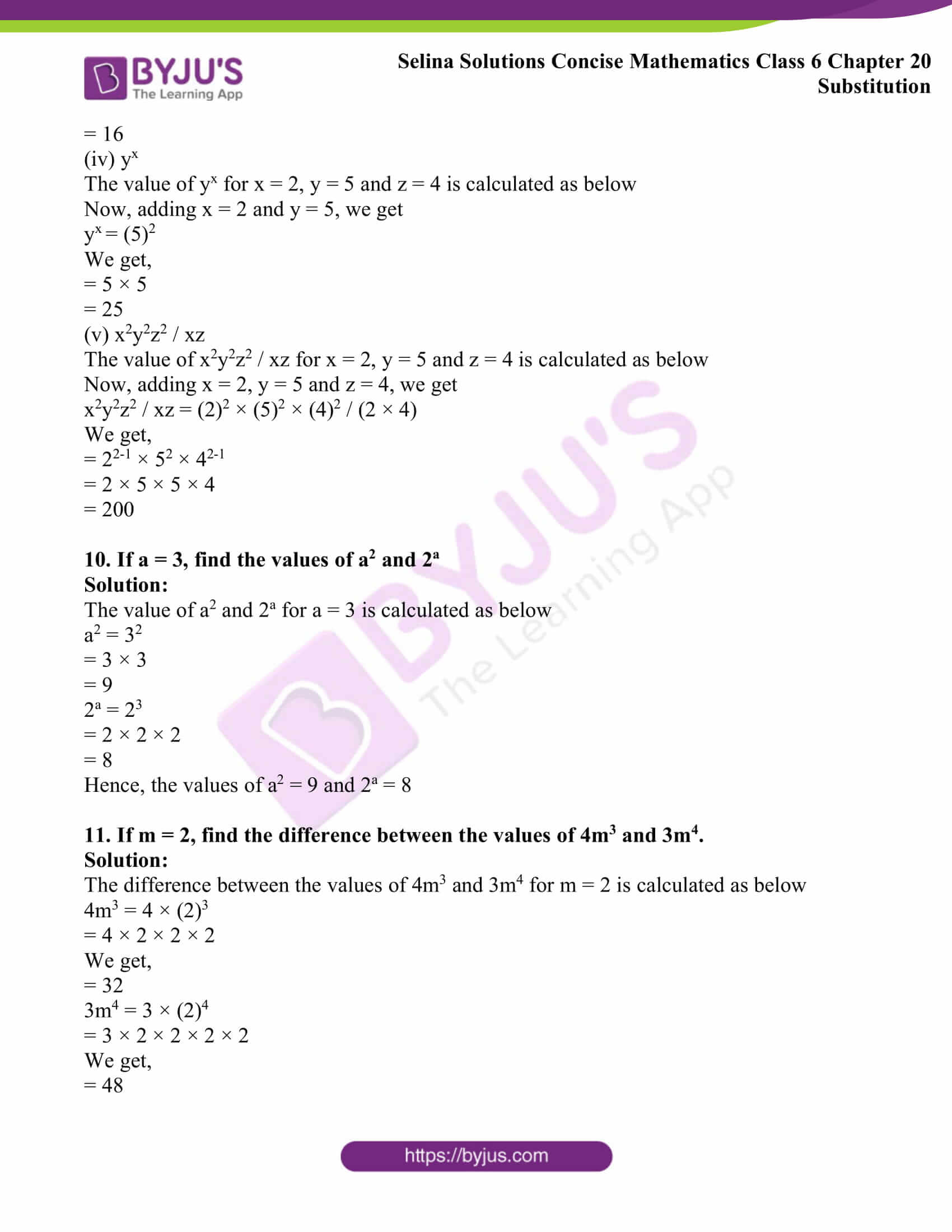### Access other exercises of Selina Solutions Concise Mathematics Class 6 Chapter 20 Substitution

Exercise 20(B) Solutions

Exercise 20(C) Solutions

### Access Selina Solutions Concise Mathematics Class 6 Chapter 20 Substitution Exercise 20(A)

#### Exercise 20(A)

1. Fill in the following blanks, when:

x = 3, y = 6, z = 18, a = 2, b = 8, c = 32 and d = 0.

(i) x + y = ……….

(ii) y – x = ……….

(iii) y / x = ………..

(iv) c ÷ b = ………..

(v) z ÷ x = ………..

Solution:

(i) x + y = ……..

The value of x + y is calculated as shown below

x + y = 3 + 6

= 9

∴ x + y = 9

(ii) y – x = ……….

The value of y – x is calculated as shown below

y – x = 6 – 3

= 3

∴ y – x = 3

(iii) y / x = ………..

The value of y / x is calculated as shown below

y / x= 6 / 3

= 2

∴ y / x = 2

(iv) c ÷ b = ………

The value of c ÷ b is calculated as shown below

c ÷ b = 32 ÷ 8

32 / 8 = 4

∴ c ÷ b = 4

(v) z ÷ x = ……….

The value of z ÷ x is calculated as shown below

z ÷ x = 18 ÷ 3

= 6

∴ z ÷ x = 6

2. Find the value of:

(i) p + 2q + 3r, when p = 1, q = 5 and r = 2

(ii) 2a + 4b + 5c, when a = 5, b = 10 and c = 20

(iii) 3a – 2b, when a = 8 and b = 10

(iv) 5x + 3y – 6z, when x = 3, y = 5 and z = 4

(v) 2p – 3q + 4r – 8s, when p = 10, q = 8, r = 6 and s = 2

Solution:

(i) p + 2q + 3r, when p = 1, q = 5 and r = 2

The value of p + 2q + 3r is calculated as shown below

p + 2q + 3r = 1 + 2 × 5 + 3 × 2

= 1 + 10 + 6

= 17

Therefore, p + 2q + 3r = 17

(ii) 2a + 4b + 5c, when a = 5, b = 10 and c = 20

The value of 2a + 4b + 5c is calculated as shown below

2a + 4b + 5c = 2 × 5 + 4 × 10 + 5 × 20

= 10 + 40 + 100

= 150

Therefore, 2a + 4b + 5c = 150

(iii) 3a – 2b, when a = 8 and b = 10

The value of 3a – 2b is calculated as shown below

3a – 2b = 3 × 8 – 2 × 10

= 24 – 20

= 4

Therefore, 3a – 2b = 4

(iv) 5x + 3y – 6z, when x = 3, y = 5 and z = 4

The value of 5x + 3y – 6z is calculated as shown below

5x + 3y – 6z = 5 × 3 + 3 × 5 – 6 × 4

= 15 + 15 – 24

= 30 – 24

= 6

Therefore, 5x + 3y – 6z = 6

(v) 2p – 3q + 4r – 8s, when p = 10, q = 8, r = 6 and s = 2

The value of 2p – 3q + 4r – 8s is calculated as shown below

2p – 3q + 4r – 8s = 2 × 10 – 3 × 8 + 4 × 6 – 8 × 2

= 20 – 24 + 24 – 16

= 4

Therefore, 2p – 3q + 4r – 8s = 4

3. Find the value of:

(i) 4pq × 2r, when p = 5, q = 3 and r = 1 / 2

(ii) yx / z, when x = 8, y = 4 and z = 16

(iii) (a + b – c) / 2a, when a = 5, b = 7 and c = 2

Solution:

(i) 4pq × 2r, when p = 5, q = 3 and r = 1 / 2

The value of 4pq × 2r is calculated as below

4pq × 2r = 4 × 5 × 3 × 2 × (1 / 2)

= 4 × 5 × 3

= 60

∴ 4pq × 2r = 60

(ii) yx / z, when x = 8, y = 4 and z = 16

The value of yx / z is calculated as below

yx / z = (4 × 8) / 16

= 32 / 16

= 2

∴ yx / z = 2

(iii) (a + b – c) / 2a, when a = 5, b = 7 and c = 2

The value of (a + b – c) / 2a is calculated as below

(a + b – c) / 2a = (5 + 7 – 2) / (2 × 5)

= 10 / 10

= 1

4. If a = 3, b = 0, c = 2 and d = 1, find the value of:

(i) 3a + 2b – 6c + 4d

(ii) 6a – 3b – 4c – 2d

(iii) ab – bc + cd – da

(iv) abc – bcd + cda

(v) a2 + 2b2 – 3c2

Solution:

(i) 3a + 2b – 6c + 4d

The value of 3a + 2b – 6c + 4d is calculated as shown below

3a + 2b – 6c + 4d = 3 × 3 + 2 × 0 – 6 × 2 + 4 × 1

On further calculation, we get

= 9 + 0 – 12 + 4

= 9 – 12 + 4

= 13 – 12

= 1

Therefore, 3a + 2b – 6c + 4d = 1

(ii) 6a – 3b – 4c – 2d

The value of 6a – 3b – 4c – 2d is calculated as shown below

6a – 3b – 4c – 2d = 6 × 3 – 3 × 0 – 4 × 2 – 2 × 1

On further calculation, we get

= 18 – 0 – 8 – 2

= 18 – 10

= 8

Therefore, 6a – 3b – 4c – 2d = 8

(iii) ab – bc + cd – da

The value of ab – bc + cd – da is calculated as shown below

ab – bc + cd – da = 3 × 0 – 0 × 2 + 2 × 1 – 1 × 3

On further calculation, we get

= 0 – 0 + 2 – 3

= 2 – 3

= – 1

Therefore, ab – bc + cd – da = – 1

(iv) abc – bcd + cda

The value of abc – bcd + cda is calculated as shown below

abc – bcd + cda = 3 × 0 × 2 – 0 × 2 × 1 + 2 × 1 × 3

On further calculation, we get

= 0 – 0 + 6

= 6

Therefore, abc – bcd + cda = 6

(v) a2 + 2b2 – 3c2

The value of a2 + 2b2 – 3c2 is calculated as shown below

a2 + 2b2 – 3c2 = (3)2 + 2 × (0)2 – 3 × (2)2

On further calculation, we get

= 9 + 0 – 12

= 9 – 12

= – 3

Therefore, a2 + 2b2 – 3c2 = – 3

5. Find the value of 5x2 – 3x + 2, when x = 2

Solution:

The value of 5x2 – 3x + 2 when x = 2 is calculated as below

5x2 – 3x + 2 = 5 × (2)2 – 3 × (2) + 2

On simplification, we get

= 5 × 4 – 3 × 2 + 2

= 20 – 6 + 2

= 22 – 6

= 16

Hence, the value of 5x2 – 3x + 2 when x = 2 is 16

6. Find the value of 3x3 – 4x2 + 5x – 6, when x = – 1

Solution:

The value of 3x3 – 4x2 + 5x – 6 when x = -1 is calculated as below

3x3 – 4x2 + 5x – 6 = 3 × (- 1)3 – 4 × (- 1)2 + 5 × (- 1) – 6

On simplification, we get

= – 3 – 4 – 5 – 6

= – 18

Hence, the value of 3x3 – 4x2 + 5x – 6 when x = – 1 is – 18

7. Show that the value of x3 – 8x2 + 12x – 5 is zero, when x = 1

Solution:

The value of x3 – 8x2 + 12x – 5 = 0 when x = 1 is calculated as below

x3 – 8x2 + 12x – 5 = (1)3 – 8 × (1)2 + 12 × (1) – 5

On simplification, we get

= 1 – 8 × 1 + 12 × 1 – 5

= 1 – 8 + 12 – 5

= 0

The value of x3 – 8x2 + 12x – 5 = 0 when x = 1

Hence, proved

8. State true and false:

(i) The value of x + 5 = 6, when x = 1

(ii) The value of 2x – 3 = 1, when x = 0

(iii) (2x – 4) / (x + 1) = -1, when x = 1

Solution:

(i) The value of x + 5 = 6, when x = 1

The value of x + 5 = 6 for x = 1 is calculated as below

x + 5 = 6

Adding the value of x = 1, we get

1 + 5 = 6

6 = 6

Therefore, the given statement is true

(ii) The value of 2x – 3 = 1, when x = 0

The value of 2x – 3 = 1 for x = 0 is calculated as below

2x – 3 = 1

Adding the value of x = 0, we get

2(0) – 3 = 1

0 – 3 = 1

– 3 = 1

Therefore, the given statement is false

(iii) (2x – 4) / (x + 1) = -1, when x = 1

The value of (2x – 4) / (x + 1) = -1 for x = 1 is calculated as below

(2x – 4) / (x + 1) = -1

Adding x = 1, we get

2(1) – 4 / (1 + 1) = – 1

– 2 / 2 = – 1

– 1 = – 1

Therefore, the given statement is true

9. If x = 2, y = 5 and z = 4, find the value of each of the following:

(i) x / 2x2

(ii) xz / yz

(iii) zx

(iv) yx

(v) x2y2z2 / xz

Solution:

(i) x / 2x2

The value of x / 2x2 for x = 2, y = 5 and z = 4 is calculated as below

x / 2x2

Now, adding x = 2, y = 5 and z = 4, we get

x / 2x2 = 2 / 2(2)2

On calculation, we get

= 2 / 8

= 1 / 4

(ii) xz / yz

The value of xz / yz for x = 2, y = 5 and z = 4 is calculated as below

xz / yz

Now, adding x = 2, y = 5 and z = 4, we get

xz / yz = (2) (4) / (5) (4)

On calculation, we get

= 8 / 20

= 2 / 5

(iii) zx

The value of zx for x = 2, y = 5 and z = 4 is calculated as below

Now, adding x = 2 and z = 4, we get

zx = (4)2

We get

= 4 × 4

= 16

(iv) yx

The value of yx for x = 2, y = 5 and z = 4 is calculated as below

Now, adding x = 2 and y = 5, we get

yx = (5)2

We get,

= 5 × 5

= 25

(v) x2y2z2 / xz

The value of x2y2z2 / xz for x = 2, y = 5 and z = 4 is calculated as below

Now, adding x = 2, y = 5 and z = 4, we get

x2y2z2 / xz = (2)2 × (5)2 × (4)2 / (2 × 4)

We get,

= 22-1 × 52 × 42-1

= 2 × 5 × 5 × 4

= 200

10. If a = 3, find the values of a2 and 2a

Solution:

The value of a2 and 2a for a = 3 is calculated as below

a2 = 32

= 3 × 3

= 9

2a = 23

= 2 × 2 × 2

= 8

Hence, the values of a2 = 9 and 2a = 8

11. If m = 2, find the difference between the values of 4m3 and 3m4.

Solution:

The difference between the values of 4m3 and 3m4 for m = 2 is calculated as below

4m3 = 4 × (2)3

= 4 × 2 × 2 × 2

We get,

= 32

3m4 = 3 × (2)4

= 3 × 2 × 2 × 2 × 2

We get,

= 48

Therefore, the difference of 4m3 and 3m4 is calculated as,

3m4 – 4m3 = 48 – 32

= 16

Hence, the difference between the given values is 16